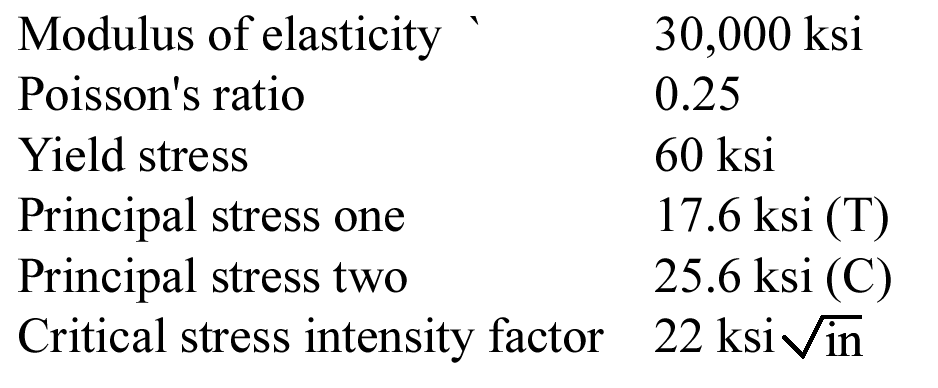# 2.2 – MATERIAL MODELS

Using the information below, choose the correct answer to the closest 3 significant figures. For questions 1 through 5, assume plane stress. For questions 6 through 10, assume plane strain.Name Email
1.

Assume plane stress:
The principal strain one is

2.

Assume plane stress:
The principal strain three is

3.

Assume plane stress:
The factor of safety to avoid yielding using maximum shear stress theory is

4.

Assume plane stress:
The factor of safety to avoid yielding using maximum octahedral shear stress theory is

5.

Assume plane stress:
The critical crack length in Mode I is

6.

Assume plane strain:
The principal stress three is

7.

Assume plane strain
The principal strain one is

8.

Assume plain strain:
The factor of safety to avoid yielding using maximum shear stress theory is

9.

Assume plane strain:
The von-Mises stress is

10.

Assume plane strain:
The factor of safety to avoid yielding using maximum octahedral shear stress theory is